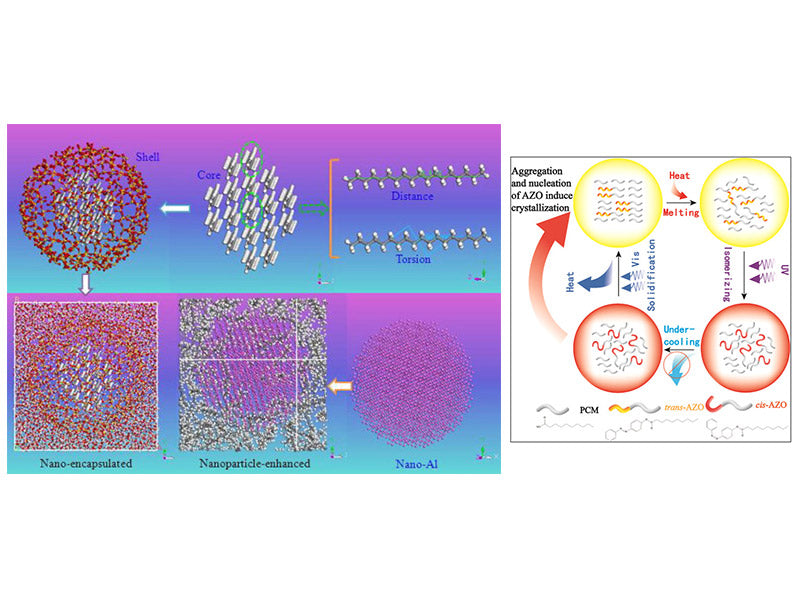Due to the differences in experimental methods and testing methods, such as the dispersion uniformity difference when adding high thermal conductivity particles, in the study of PCM(phase change materials) to enhance heat transfer, different researchers will have certain differences in the research on the thermophysical properties of the same material. Sari and Karaipekli, Xia et al. and Zhong et al. all experimentally investigated the thermal conductivity of paraffin and expanded graphite/paraffin composites. Among them, the research results of Sari and Karaipekli are that after adding 2%, 4%, 7% and 10% of expanded graphite, respectively, the thermal conductivity of PCM is 0.40W·m-1·K-1, 0.52W·m-1·K-1, 0.68W·m-1·K-1 and 0.82W·m-1·K-1, respectively; after adding the same proportion of expanded graphite, in the experimental results of Xia et al, the corresponding thermal conductivity of PCM is about 0.50W·m-1·K-1, 1.28W·m-1·K-1, 3.03W·m-1·K-1 and 3.88W·m-1·K-1, respectively; for pure paraffin without adding expanded graphite, their test results were 0.22W·m-1·K-1 and 0.305W·m-1·K-1, respectively. Zhong et al. compressed expanded graphite to several different bulk densities, and then mixed it with paraffin, and found that with the change of bulk density of expanded graphite, the thermal conductivity of composite PCM could be increased by 28-180 times. Due to factors such as the accuracy of the test instrument, the amount and shape of the test sample, etc., the experimental results may also be different. For other thermophysical properties of PCM, such as using differential scanning calorimetry (DSC) to test the latent heat of phase transition, phase transition temperature and other parameters of composite PCM, due to the small sample size and different test conditions, the results obtained may also vary. In addition, the experimental equipment involved in some experiments is expensive and the experimental cost is high. Therefore, it is very necessary to obtain the thermophysical properties of PCM by a research method that can complement the experiment and is not limited by objective environmental conditions, and has certain guiding significance for the material design of new PCM.

According to the method and theory of molecular dynamics simulation, combined with the application of PCM in the thermal management of power battery, the static and flow characteristics of PCM and the battery in different ways are studied. The following mainly introduces the method and theory of molecular dynamics simulation, to pave the way for the related research work on the specific heat and thermal conductivity of elemental alkane, the diffusion characteristics of high thermal conductivity nano metal particles in alkane and the self-diffusion characteristics of alkane-based phase change capsule heat transfer medium by establishing molecular models of different PCM systems and using molecular dynamics simulation.

Molecular dynamics simulation is through the interaction potential function between the microscopic particles (molecules, atoms, ions, collectively referred to as molecules in molecular dynamics simulation) that make up the system, according to the Newtonian law of motion of classical mechanics or quantum mechanics method, solve the equation of motion, study the law of motion of microscopic particles, conduct statistics on the microscopic state of the system, and then obtain the thermodynamic quantity and other macroscopic properties of the system. For a moving system containing N molecules or atoms, the energy of the system is the sum of the kinetic energy of the molecules in the system and the total potential energy, and the total potential energy is a function of the position of each atom in the molecule. According to classical mechanics, the force on any atom i in the system can be expressed asFrom Newton's second law, the acceleration of atom i can be obtained asBy integrating the time in the equation of Newton's law of motion, the velocity and position of atom i after time t can be predicted, which are expressed as follows:In the formula, vi and r represent the velocity and position of the particle respectively; the superscript “0” represents the initial value of each physical quantity. By solving the above equations, parameters such as the position, velocity and acceleration of the molecular motion in the system at each time can be obtained. The key to molecular dynamics simulation is to solve the above-mentioned Newton's equation of motion, generally using Velocity-Verlet algorithm that can simultaneously obtain position, acceleration and velocity. According to this algorithm, the position, acceleration and velocity of atoms at time t+Δt can be obtained, which can be expressed as follows: